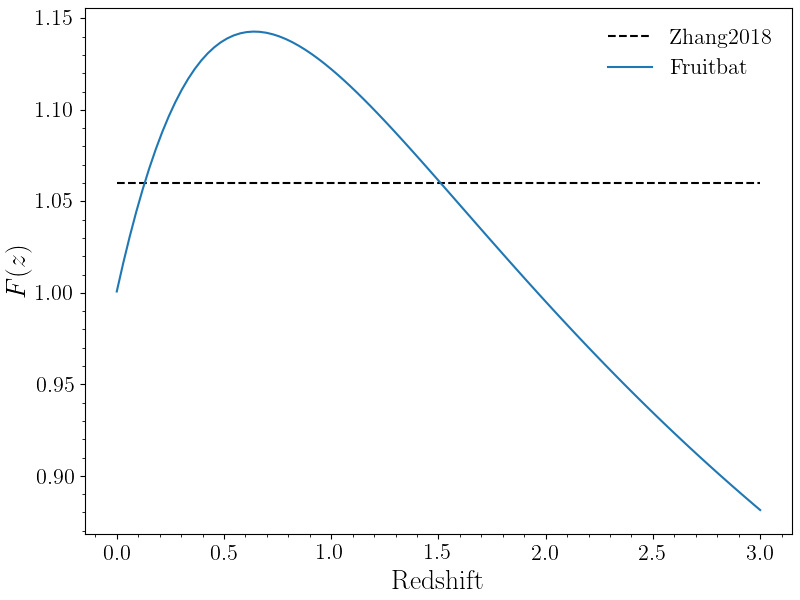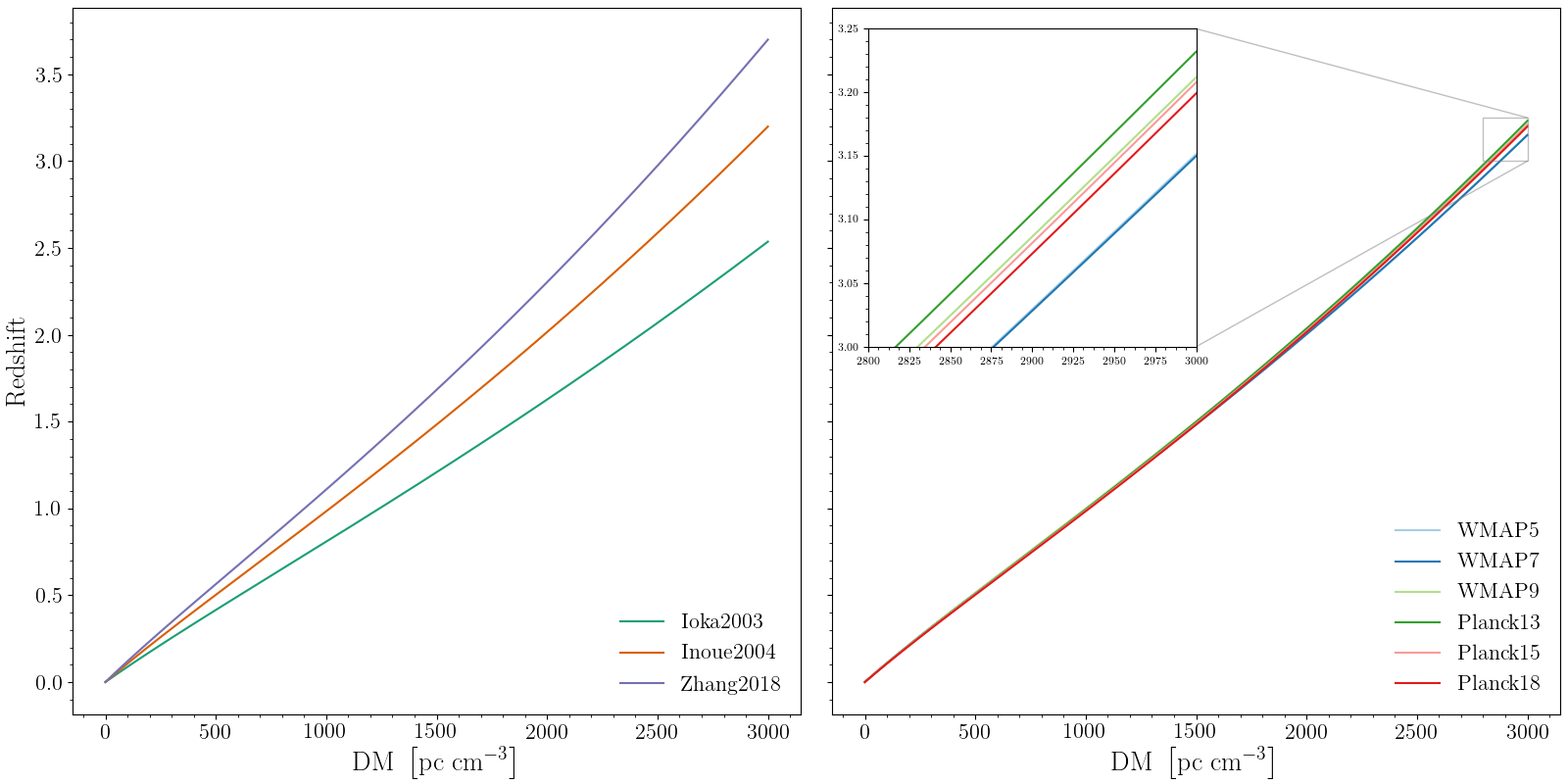# Methods and Cosmology¶

When using fruitbat there are different methods and cosmologies built-in from which you can choose. Below is a brief description of how each method is calculated and the parameters for each cosmology.

## Methods¶

Currently there are three built-in dispersion measure-redshift relations. In fruitbat these are referred to as ‘methods’. To calculate the look-up tables fruitbat uses the equations described below for each method to calculate the dispersion measre ($$\rm{DM}$$) for a given redshift ($$z$$).

### F(z) Integral¶

The methods 'Ioka2003', 'Inoue2004', 'Zhang2018' all have a common integral $$F(z)$$.

$F(z) = \int_0^z \frac{1 + z}{\left[\Omega_m(1+z)^3 + \Omega_{\Lambda} (1+z)^{3(1-w)} \right]^{1/2}}dz$

Where $$z$$ is the redshift, $$\Omega_m$$ is the cosmic matter density, $$\Omega_{\Lambda}$$ is cosmic dark energy density and $$w$$ characterises the dark energy equation of state. This is typically assumed to have a constant value of $$\sim 1.06$$ which introduces an error of approximately 6% for $$z < 2$$.

The lookup tables in fruitbat explicitly solve this integral for each redshift to when calculating a dispersion measure. See the Figure below for a comparison between the assumed value and the integral in fruitbat.### Ioka 2003¶

The Ioka (2003) method assumes that all baryons in the Universe are fully ionised and that there is a 1-to-1 relation between eletrons and baryons. i.e. The number is free electrons in the Universe is the same as the number of baryons. The DM at a given redshift using the Ioka method is calculated as follows:

$\begin{split}\mathrm{DM} &= \frac{3 c H_0 \Omega_b}{8 \pi G m_p} F(z) \\ &= 1108 \mathrm{pc\ cm^{-3}} F(z)\end{split}$

Where $$c$$ is the speed of light, $$H_0$$ is the Hubble constant, $$\Omega_b$$ is the cosmic baryon density, $$G$$ is the gravitational constant, $$m_p$$ is the mass of the proton and $$F(z)$$ is the integral defined earlier.

### Inoue 2004¶

The Inoue (2004) method assumes that hydrogen is fully ionised and helium is singly ionised. THe DM at a given redshift using the Inoue method is calculated as follows.

$\begin{split}\mathrm{DM} &= 9.2 \times 10^{-10} c H_0 \Omega_b F(z) \\ &= 908 \mathrm{pc\ cm^{-3}} F(z)\end{split}$

Where $$c$$ is the speed of light, $$H_0$$ is the Hubble constant, $$\Omega_b$$ is the cosmic baryon density and $$F(z)$$ is the integral defined earlier. The factor of $$9.2 \times 10^{-10}$$ comes estimating the number of free electrons at high redshifts from models of reionisation.

### Zhang 2018¶

The Zhang (2018) method assumes that all baryons in the Universe are fully ionised and that there is a 0.875-to-1 ratio between eletrons and baryons, and that 85% of baryons are in the intergalactic medium. The DM at a given redshift using the Zhang method is calculated as follows:

$\begin{split}\mathrm{DM} &= \frac{3 c H_0 \Omega_b \chi f_{igm}}{8 \pi G m_p} F(z) \\ &= 805 \mathrm{pc\ cm^{-3}} \left(\frac{f_{igm}}{0.83}\right) \left(\frac{\chi}{0.875}\right) F(z)\end{split}$

Where $$c$$ is the speed of light, $$H_0$$ is the Hubble constant, $$\Omega_b$$ is the cosmic baryon density, $$G$$ is the gravitational constant, $$m_p$$ is the mass of the proton, $$\chi$$ is the free electron per baryon in the Universe, $$f_{igm}$$ is the fraction of baryons in the intergalactic medium and $$F(z)$$ is the integral defined earlier.

$$\chi$$ is calculated as follows:

$\chi(z) = \frac{3}{4} y_1 \chi_{e, H}(z) + \frac{1}{8} y_2 \chi_{e, He}(z)$

Where $$\chi_{e, H}$$ and $$\chi_{e, He}$$ denote the ionisation fraction of hydrogen and helium respectively and $$y_1 \sim y_2 \sim 1$$ denote the possible slight deviation from the 3/4 - 1/4 split of hydrogen and helium abundance in the Universe. Assuming that hydrogen and helium are both ionised, then $$\chi(z) \sim 0.875$$.

## Cosmology¶

Each method in fruitbat has a list of pre-calculated lookup tables with different cosmologies. The table below lists the parameters that are used for each cosmology.

Cosmological Parameters
Keyword $$H_0$$ $$\Omega_b$$ $$\Omega_m$$ $$\Omega_\Lambda$$ $$w$$
'WMAP5' $$70.2$$ $$0.0459$$ $$0.277$$ $$0.723$$ -1
'WMAP7' $$70.4$$ $$0.0455$$ $$0.272$$ $$0.728$$ -1
'WMAP9' $$69.32$$ $$0.04628$$ $$0.2865$$ $$0.7134$$ -1
'Planck13' $$67.77$$ $$0.0483$$ $$0.3071$$ $$0.6914$$ -1
'Planck15' $$67.74$$ $$0.0486$$ $$0.3075$$ $$0.6910$$ -1
'Planck18' $$67.66$$ $$0.04897$$ $$0.3111$$ $$0.6874$$ -1

Below is a figure comparing the different methods and cosmologies in fruitbat. The left figure shows how the different methods compare assuming a 'Planck18' cosmology.. The right figure shows how the 'Inoue2004' method changes with different assumed cosmologies.